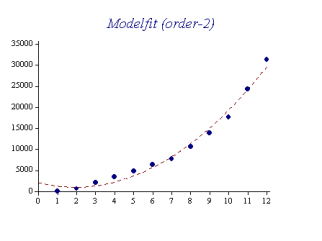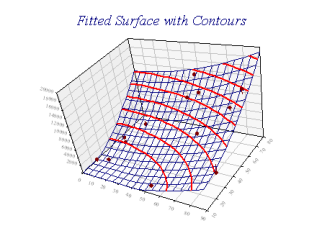SharpPlot Tutorials > Style Examples > Model fit

## Model fit

A simple regression model is fitted to the data.`sp.LineGraphStyle = LineGraphStyles.ModelFit;`

The default is a linear ‘straight-line’ fit but you can use the Order of Fit setting to fit models up to quartics. The line may be annotated with the regression coefficients. The ‘zeroth’ coefficient is the intercept value, and there will be as many further coefficients as the order of fit.

The model line consumes one line colour, style, width, and key in the cycles, in the order (data, trend, model).

### Fitting 3D data

If the modelfit style is used with the cloud chart, the fit is made against both X and Y axes, and the order of fit may be set independently for either.Here, the model is quadratic in both X and Y. Contour lines are shown, and risers have been used to show the residual values in the Z-direction.